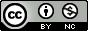Scientific journal NRU ITMO
Series "Refrigeration and Air Conditioning"
Registration certificate ЭЛ № ФС 77 – 55410 от 17.09.2013
зарегистрировано в Федеральной службе по надзору в сфере связи, информационных технологий и массовых коммуникаций
ISSN:2310-1148
Publications

December 2014 (published: 23.12.2014)

Number 3(16)

Home > Issue > Calculation of a line of elasticity perfluoropropane in MATHCAD

## Calculation of a line of elasticity perfluoropropane in MATHCAD

Builtequation of the line of elasticity, taking into account the requirements of large-scale hypothesis and the behavior of the saturated vapor pressure near the triple point. In contrast to known models describing the line of elasticity in the temperature range from the triple point to the critical, the proposed model describes a line of elasticity in the general vicinity of the critical point, in accordance with the requirements of the modern theory of critical phenomena. Based on the proposed line equation of elasticity throughout the specified temperature range designed for perfluoropropene detailed table comprising values of the pressure, the first and second derivatives of the pressure on the phase equilibrium line as a function of temperature.The value calculated on the obtained equations are not inferior to the currently used tables in accuracy to describe the experimental data. The relative deviation from the experimental and tabular data presented in the figure. The paper contains the values of all parameters included in the equation. Also the dependences for calculation of first and second derivatives line of elasticity. The obtained equation can be used to calculate the exact thermodynamic tables on the line of phase equilibrium and to obtain the support of the array points in the calculation of the coefficients of a large-scale and fundamental equations of state. Possible to usethe resulting equation in the article to describe the elastic line of other technologically important materials used in refrigeration and food industries.
Read the full articleKeywords: lineelasticity, pressure, perfluoropropane, R218, critical exponents.